Documentation

## Modeling and Analyzing Polarization

This example introduces the basic concept of polarization. It shows how to analyze the polarized field and model the signal transmission between polarized antennas and targets using Phased Array System Toolbox™.

### Polarization of an Electromagnetic Field

The electromagnetic field generated by an antenna is orthogonal to the propagation direction in the far field. The field can be pointing to any direction in this plane, and therefore can be decomposed into two orthogonal components. Theoretically, there are an infinite number of ways to define these two components, but most often, one uses either (H,V) set or (L,R) set. (H,V) stands for horizontal and vertical, which can be easily pictured as x and y component; while (L,R) stands for left and right circular. It may be difficult to imagine that a vector in space can have a circular component in it, the secret lies in the fact that each component can be a complex number, which greatly increases the complexity of the trace of such a vector.

Let's look at several simple examples. The time varying field can be written as

``

where

``

are the two components in phasor representation.andare the unit vector of h and v axes, respectively.

The simplest case is probably a linear polarization, which happens when the two components are always in phase. Assume,

the field can be represented by a vector of [1;1]. The polarization for such a field looks like

```fv = [1;1]; helperPolarizationView(fv) ```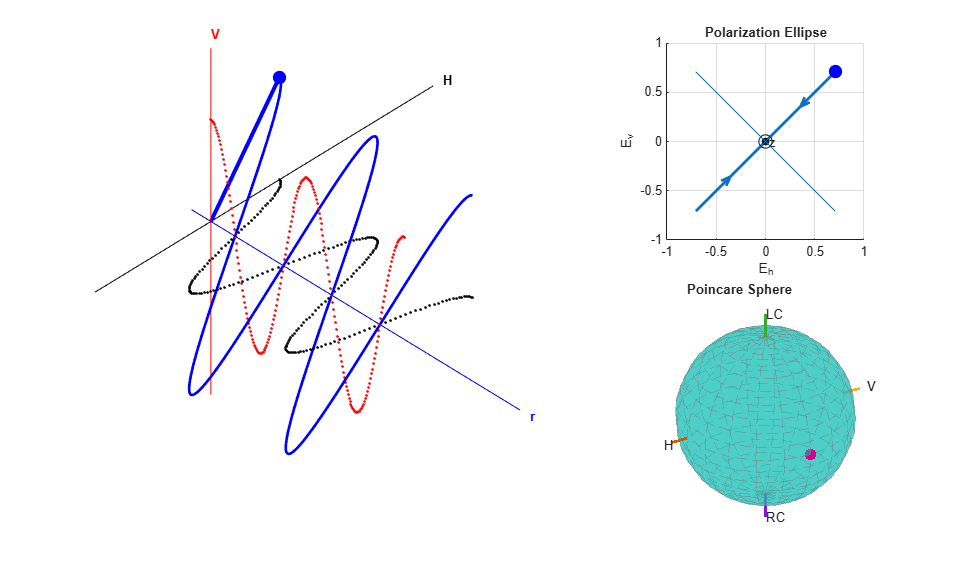From the figure, it is clear that the combined polarization is along the 45 degrees diagonal.

The plot in the upper right portion of the figure is often referred to as the polarization ellipse. It is the projection of the combined field trace on the H-V plane. The polarization ellipse is often characterized by two angles, the tilt angle (also known as orientation angle)and the ellipticity angle. In this case, the tilt angle is 45 degrees and the ellipticity angle is 0. The dot on the ellipse shows how the combined field moves along the trace on the H-V plane while time passes.

A polarized field can also be represented by Stokes vector, which is a length-4 vector. The corresponding Stokes vector of the linear polarization, [1;1], is given by

```s = stokes(fv) ```
```s = 2 0 2 0 ```

Note that all 4 entries in the vector are real numbers. In fact, all these entries are measurable. In addition, it can be shown that the four quantities always satisfy the following equation.

Therefore, each set of Stokes can be considered as a point on a sphere. Such a sphere is referred to as a Poincare sphere. The Poincare sphere for the above field is shown in the bottom right portion of the figure.

Next is a circular polarized field, where

``
```fv = [1;1i]; helperPolarizationView(fv) ```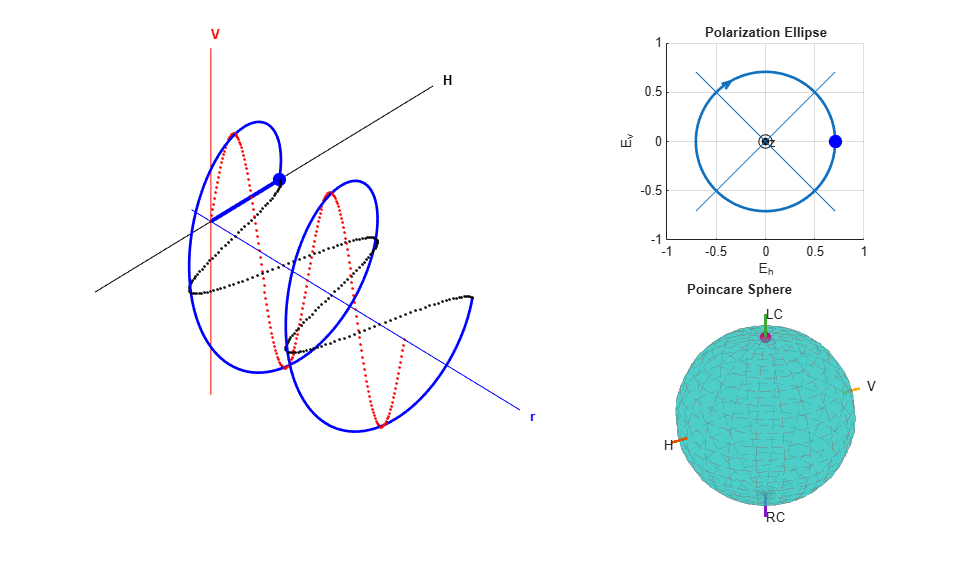The figure shows that the trace of the combined field is a circle. Both the polarization ellipse and the Poincare sphere shows that the field is left circularly polarized.

In general, the trace of a field is an ellipse, as shown below

```fv = [2+1i;1-1i]; helperPolarizationView(fv) ```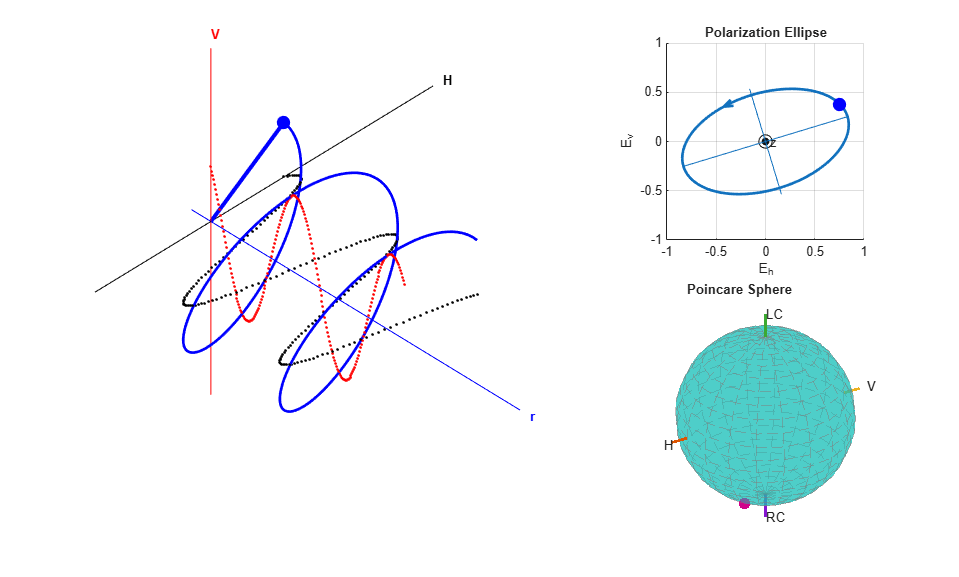### Polarization of an Antenna

The polarization of an antenna is defined as the polarization of the field transmitted by the antenna regardless whether it's in the transmitting or receiving mode. However, as mentioned earlier, the polarization is defined in the plane that is orthogonal to the propagation direction. Therefore, it is defined in the local coordinate system of each propagation direction, as shown in the following diagram.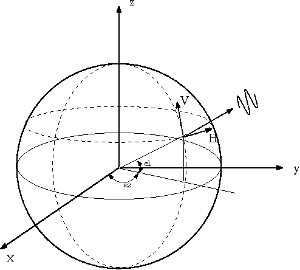Some antennas have a structure that determines its polarization, such as a dipole. The dipole antenna has a polarization that is parallel to its orientation. Assuming the frequency is 300 MHz, for a vertical short dipole, the polarization response at boresight, i.e., 0 degrees azimuth and 0 degrees elevation, is given by

```antenna = phased.ShortDipoleAntennaElement('AxisDirection','Z'); fc = 3e8; resp = antenna(fc,[0;0]) ```
```resp = struct with fields: H: 0 V: -1.2247 ```

Note that the horizontal component is 0. If we change the orientation of the dipole antenna to horizontal, the vertical component becomes 0.

```antenna = phased.ShortDipoleAntennaElement('AxisDirection','Y'); resp = antenna(fc,[0;0]) ```
```resp = struct with fields: H: -1.2247 V: 0 ```

### Polarization Loss

When two antennas form a transmit/receive pair, their polarizations could affect the received signal power. Therefore, to collect a signal with maximum power possible, the receive antenna's polarization has to match the transmit antenna's polarization. The polarization matching factor can be measured as

``

whereandrepresent the normalized polarization states of the transmit and receive antenna, respectively.

Assume both transmit and receive antennas are short dipoles. The transmit antenna sits at the origin and the receive antenna at location (100,0,0). First, consider the case where both antennas are along Y axis and face each other. This is the scenario where the two antennas are matched in polarization.

```pos_r = [100;0;0]; lclaxes_t = azelaxes(0,0); % transmitter coordinate system lclaxes_r = azelaxes(180,0); % receiver faces transmitter ang_t = [0;0]; % receiver at transmitter's boresight ang_r = [0;0]; % transmitter at receiver's boresight txAntenna = phased.ShortDipoleAntennaElement('AxisDirection','Z'); resp_t = txAntenna(fc,ang_t); rxAntenna = phased.ShortDipoleAntennaElement('AxisDirection','Z'); resp_r = rxAntenna(fc,ang_r); ploss = polloss([resp_t.H;resp_t.V],[resp_r.H;resp_r.V],pos_r,lclaxes_r) ```
```ploss = 0 ```

The loss is 0dB, indicating that there is no loss due to polarization mismatch. The section below shows the effect with a simulated signal.

```% Signal simulation [x,t] = helperPolarizationSignal; % Create radiator and collector radiator = phased.Radiator('Sensor',txAntenna,'Polarization','Combined',... 'OperatingFrequency',fc,'PropagationSpeed',3e8); collector = ... phased.Collector('Sensor',rxAntenna,'Polarization','Combined',... 'OperatingFrequency',fc,'PropagationSpeed',3e8); % Signal transmission and reception xt = radiator(x,ang_t,lclaxes_t); y = collector(xt,ang_r,lclaxes_r); helperPolarizationSignalPlot(t,x,y,'vertically') ```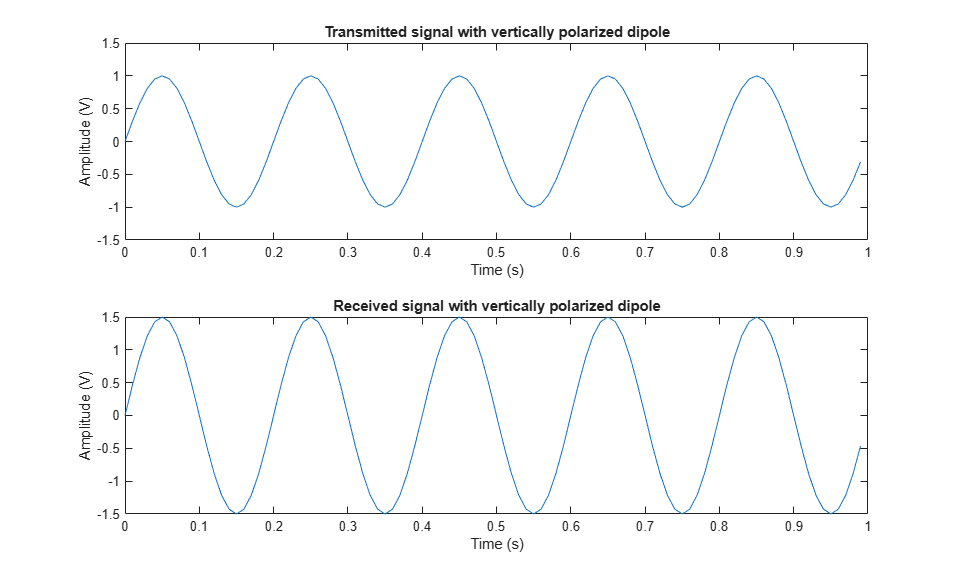The figure shows that the signal is received with no loss. Each short dipole antenna provides a gain of 1.76 dB, so the received signal is 1.5 times stronger than the transmitted signal.

If instead a horizontally polarized antenna is used to receive the signal, the two antennas are now orthogonal in polarization and as a result, no power will be delivered to the received antenna. The polarization loss can be found by

```rxAntenna = phased.ShortDipoleAntennaElement('AxisDirection','Y'); resp_r = rxAntenna(fc,ang_r); ploss = polloss([resp_t.H;resp_t.V],[resp_r.H;resp_r.V],pos_r,lclaxes_r) ```
```ploss = Inf ```

This process can be better understood using the following diagram.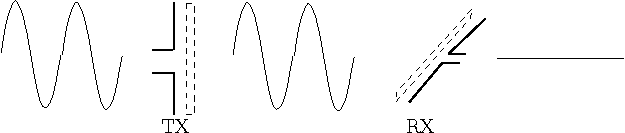As the diagram shows, the polarization of an antenna can be seen as a filter blocking out any polarized wave that is orthogonal to the antenna's own polarization state.

As expected, the signal simulation shows that the received signal is 0.

```collector = ... phased.Collector('Sensor',rxAntenna,'Polarization','Combined',... 'OperatingFrequency',fc,'PropagationSpeed',3e8); % Signal transmission and reception xt = radiator(x,ang_t,lclaxes_t); y = collector(xt,ang_r,lclaxes_r); helperPolarizationSignalPlot(t,x,y,'horizontally') ```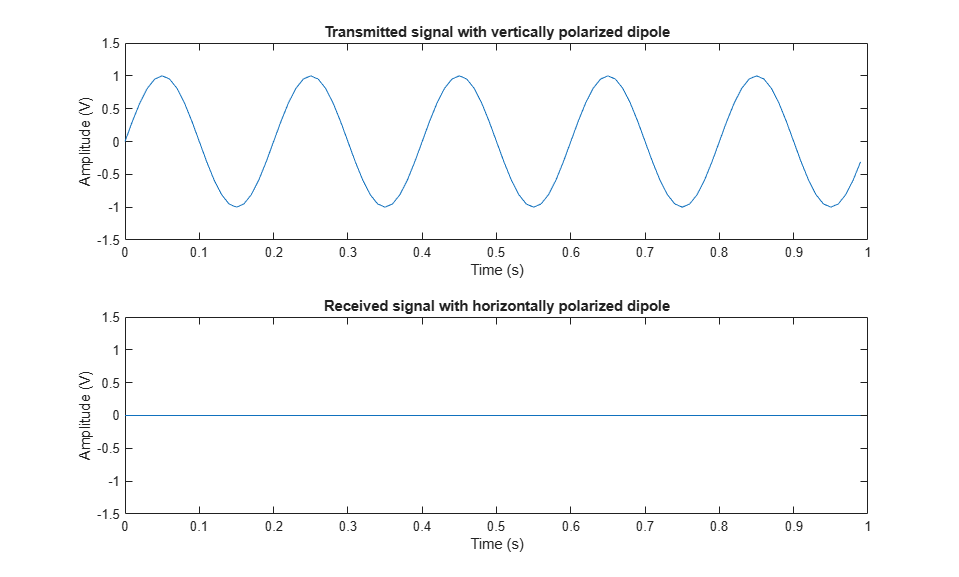One can rotate the receive antenna to get a partial match in polarization. For instance, assume the receiving antenna in the previous example is rotated 45 degrees around x axis, then the received signal is no longer 0, although not as strong as when the polarizations are matched.

```% Rotate axes lclaxes_r = rotx(45)*azelaxes(180,0); % Signal transmission and reception xt = radiator(x,ang_t,lclaxes_t); y = collector(xt,ang_r,lclaxes_r); helperPolarizationSignalPlot(t,x,y,'45 degree') ```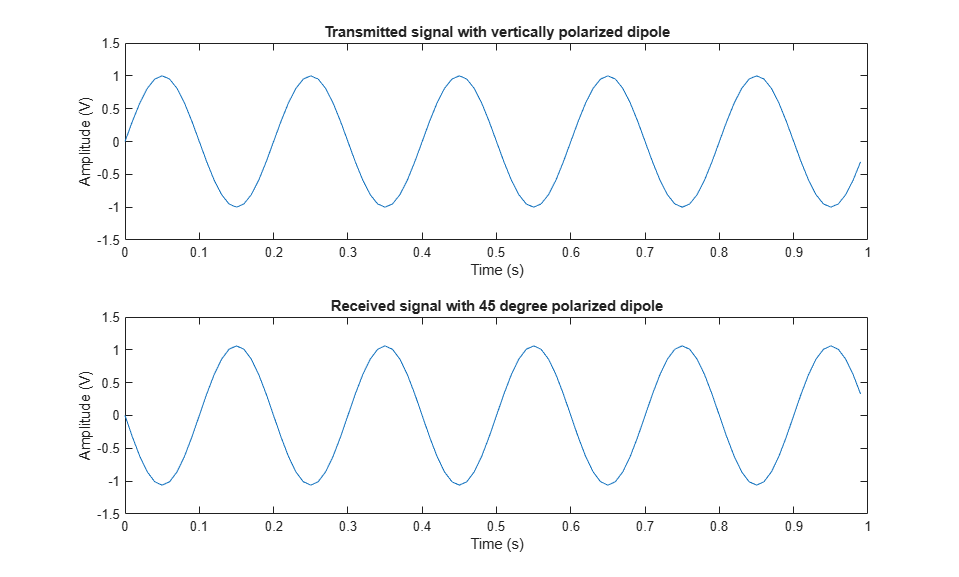The corresponding polarization loss is

```ploss = polloss([resp_t.H;resp_t.V],[resp_r.H;resp_r.V],pos_r,lclaxes_r) % measured in dB. ```
```ploss = 3.0103 ```

### Target Polarization Signature

When an electromagnetic wave hits the target, the wave will be scattered off the target and some energy will be transferred between two orthogonal polarization components. Therefore, the target scattering mechanism is often modeled by a 2x2 radar cross section (RCS) matrix (also known as scattering matrix), whose diagonal terms specify how the target scatters the energy into the original H and V polarization component and off diagonal terms specify how the target scatters the energy into the opposite polarization component.

Because the transmit and receive antennas can have any combination of polarizations, it is often of interest to look at the polarization signature for a target for different polarization configurations. The signature plots the received power under different polarizations as a function of the tilt angle and the ellipticity angle of the transmit polarization ellipse. This can also be seen as a measure of the effective RCS. Two most widely used polarization signatures (also known as polarization responses), are co-polarization (co-pol) response and cross polarization (cross-pol) response. Co-pol response uses the same polarization for both transmit and receive while the cross-pol response uses the orthogonal polarization to receive.

The simplest target is a sphere, whose RCS matrix is given by [1 0;0 1], meaning that the reflected polarization is the same as the incident polarization. The polarization signature for a sphere is given by

```s = eye(2); subplot(211); polsignature(s,'c'); subplot(212); polsignature(s,'x'); ```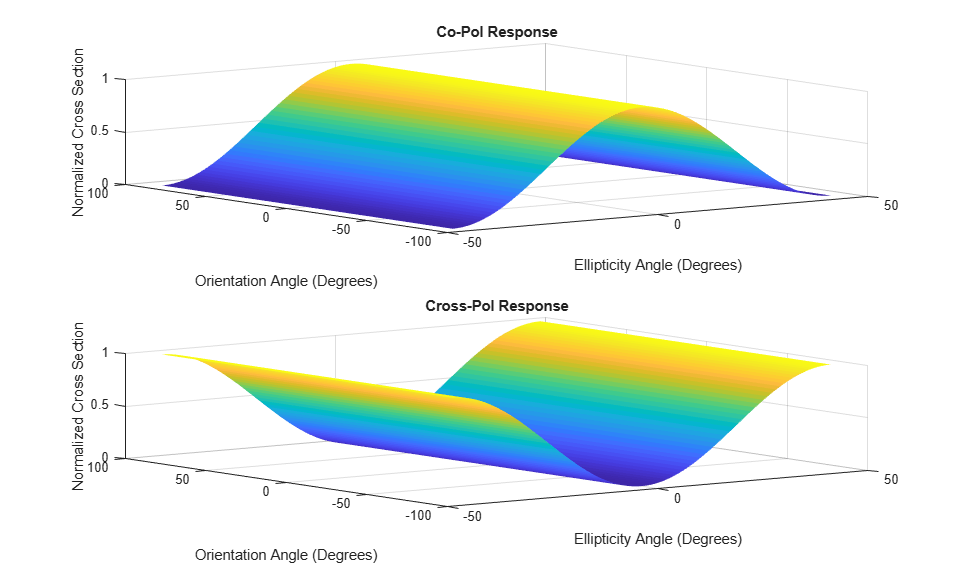From the plot, it can be seen that for such a target, a linear polarization, where the ellipticity angle is 0, generates the maximum return in a co-pol setting while a circular polarization, where the ellipticity angle is either 45 or -45 degrees, generates the maximum return in a cross-pol configuration.

A more complicated target is a dihedral, which is essentially a corner that reflects the wave twice, as shown in left side of the following sketch: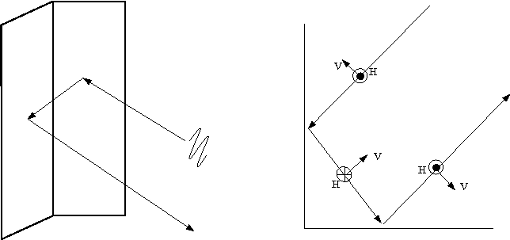The right side of the above figure shows how the polarization field changes along the two reflections. After the two reflections, the horizontal polarization component remains the same while the vertical polarization component is reversed. Hence, its cross section matrix and polarization signature are give by

```s = [1 0;0 -1]; subplot(211); polsignature(s,'c') subplot(212); polsignature(s,'x') ```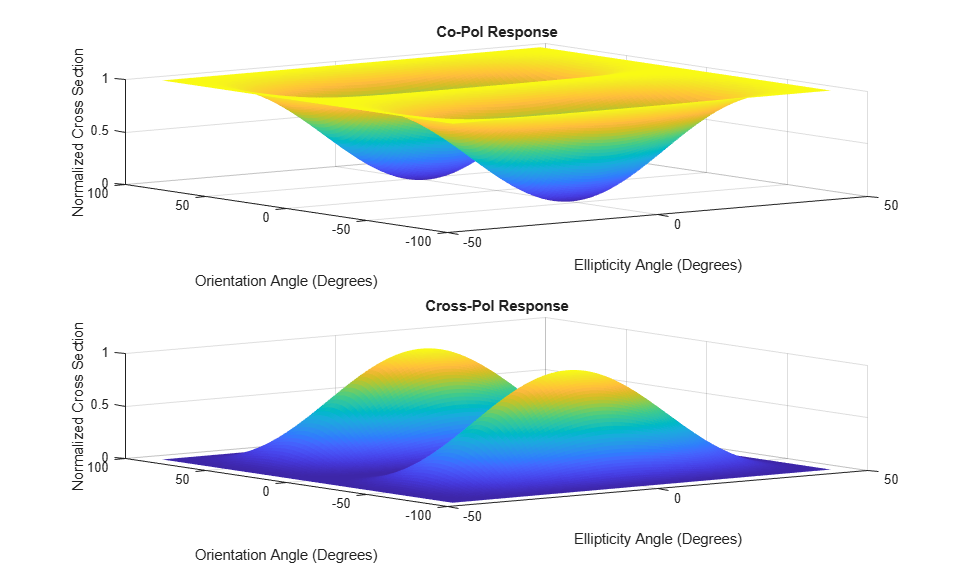The signature shows that the circular polarization works best in a co-pol setting while 45-degree linear polarization works best in a cross-pol situation.

### Simulation of Polarized Signal Propagation Using Antenna and Target

Putting everything together, the polarized signal is first transmitted by an antenna, then bounces off a target, and finally gets received at the receive antenna. Next is a simulation for this signal flow.

The simulation assumes a vertical dipole as the transmit antenna, a horizontal dipole as the receive antenna, and a target whose RCS matrix is [0 1;1 0], which flips the signal's polarization. For the illustration purpose, the propagation in free space is ignored because it does not affect the polarization. It is also assumed that the transmit antenna, the target, and the receive antenna are on a line along the transmit antenna's boresight. The local coordinate system is the same for the transmit antenna and the target. The receive antenna is facing the transmit antenna.

```% Define transmit and antenna txAntenna = phased.ShortDipoleAntennaElement('AxisDirection','Z'); rxAntenna = phased.ShortDipoleAntennaElement('AxisDirection','Y'); radiator = phased.Radiator('Sensor',txAntenna,'Polarization','Combined'); collector = phased.Collector('Sensor',rxAntenna,'Polarization','Combined'); % Simulate signal [x,t] = helperPolarizationSignal; % Incident and arriving angles ang_tx = [0;0]; ang_tgt_in = [180;0]; ang_tgt_out = [0;0]; ang_rx = [0;0]; % Local coordinate system lclaxes_tx = azelaxes(0,0); lclaxes_tgt = lclaxes_tx; lclaxes_rx = azelaxes(180,0); % Define target target = phased.RadarTarget('EnablePolarization',true,... 'Mode','Bistatic','ScatteringMatrix',[0 1;1 0]); % Simulate received signal xt = radiator(x,ang_tx,lclaxes_tx); % radiate xr = target(xt,ang_tgt_in,ang_tgt_out,lclaxes_tgt); % reflect y = collector(xr,ang_rx,lclaxes_rx); % collect helperPolarizationSignalPlot(t,x,y,'horizontally'); ```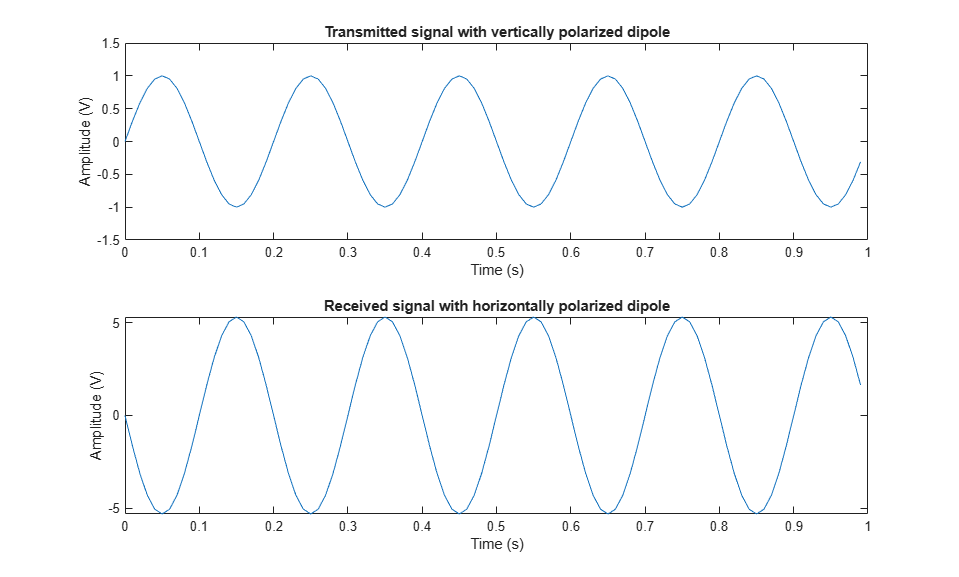Note that because the target flips the polarization components, the horizontally polarized antenna is able to receive the signal sent with a vertically polarized antenna.

### Summary

This example reviews the basic concepts of polarization and introduces how to analyze and model polarized antennas and targets using Phased Array System Toolbox.